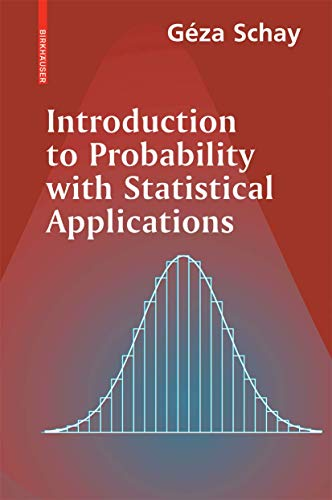# Introduction to Probability with Statistical Applications by Geza Schay

In Stock
\$27.49
+10% GST
Introduction to Probability with Statistical Applications targets non-mathematics students, undergraduates and graduates, who do not need an exhaustive treatment of the subject. The rigorous contains theorems and proofs. Linear algebra is largely avoided.
Only 1 left

## Introduction to Probability with Statistical Applications Summary

### Introduction to Probability with Statistical Applications by Geza Schay

Introduction to Probability with Statistical Applications targets non-mathematics students, undergraduates and graduates, who do not need an exhaustive treatment of the subject. The presentation is rigorous and contains theorems and proofs, and linear algebra is largely avoided so only a minimal amount of multivariable calculus is needed. The book contains clear definitions, simplified notation and techniques of statistical analysis, which combined with well-chosen examples and exercises, motivate the exposition. Theory and applications are carefully balanced. Throughout the book there are references to more advanced concepts if required.

### Why buy from World of BooksOur excellent value books literally don't cost the earthFree delivery in AustraliaEvery used book bought is one saved from landfill

## Introduction to Probability with Statistical Applications Reviews

From the reviews: "The textbook under consideration provides material for approximately a two semester introductory course in probability theory and statistics at the university level, particularly for students with non-mathematical major subjects, such as computer sciences, engineering and other natural and social sciences. It includes seven chapters in about 300 pages covering the most relevant basic fields. ... The large amount of exercises, a lot of diagrams and the mnemonic heading of each statement and definition will find a thankful audience for sure." (Michael Hogele, Zentralblatt MATH, Vol. 1135 (13), 2008) "This textbook is a classical and well-written introduction to probability theory and statistics. ... the book is written 'for an audience such as computer science students, whose mathematical background is not very strong and who do not need the detail and mathematical depth of similar books written for mathematics or statistics majors.' ... Each new concept is clearly explained and is followed by many detailed examples. ... numerous examples of calculations are given and proofs are well-detailed." (Sophie Lemaire, Mathematical Reviews, Issue 2008 m)

The Algebra of Events.- Combinatorial Problems.- Probabilities.- Random Variables.- Expectation, Variance, Moments.- Some Special Distributions.- The Elements of Mathematical Statisti.

GOR010101192
Introduction to Probability with Statistical Applications by Geza Schay
Geza Schay
Used - Very Good
Paperback
Birkhauser Boston Inc
2007-08-23
328
0817644970
9780817644970
N/A
Book picture is for illustrative purposes only, actual binding, cover or edition may vary.
This is a used book - there is no escaping the fact it has been read by someone else and it will show signs of wear and previous use. Overall we expect it to be in very good condition, but if you are not entirely satisfied please get in touch with us.